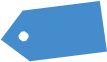# Bucc’s Put Credit Spread (Part 1)

Preface from Tim: Beloved Sloper Buccaneer was kind enough to answer my “call for content” during my most desperate hour (that is, since I’m traveling soon) and submitted a huge post which I’ve broken into several parts, not just because it’s so danged big, but also to stretch out the content for my own purposes. Thank you, Bucc!

An unconventional methodology of trading that uses Put Credit Spread option strategy (or Bull Put Spread aka Short Put Vertical) to profit from Volatility and Time Decay of SPX (S&P 500 Cash Index) underlying.

Where the river flow is the market falling, and the salmon the Premium you’re selling…

## Delta, Gamma, Theta, Vega: it’s all Greek to me.

Options are derivatives and options pricing is based on supply and demand. Greeks are used to estimate what an option price might become as underlying price, time, volatility or interest rate fluctuates. Here is a summary of how the basic Greeks affect your option positions:

Delta: how option price changes for \$1.00 move in the underlying.

Gamma: how delta changes with a \$1.00 move in the underlying.

Theta: how option prices change with 1 day in passing.

Vega: how option prices change with a 1% in volatility.

Volatility aplenty

There are two types of volatility: Historical Volatility (HV) and Implied Volatility (IV). The Implied Volatility Percentile (IV%) shows if the current Implied Volatility (IV) is high or low.

## Historical Volatility

It measures statistically an underlying’s price movement based on its historical price performance by taking a one Standard Deviation (SD) move of the underlying’s daily closing price over a given period of time. Future volatility is unknown.

Figure 1: TOS Analyze Tab of SPX bell curve with 1 SD.

Figure 2: TW SPX bell curve.

1 SD shows the expected range of SPX to stay within a certain price range. The probability of expiring within that range is about 68.27%. Furthermore, if we assume stocks have a simple normal price distribution, we can calculate what a 1 SD move for the underlying will be. On an annualized basis SPX will stay within +/- 1 SD, roughly 68% of the time.

2 SD will give you a probability of 95.45% that SPX will stay within that price range.

3 SD will give you a probability of 99.73% that SPX will stay within that price range.

## The Probability of Profit (POP)

When we sell options, we sell them at strikes that are At the Money (ATM where the underlying price is trading) or Out of the Money (OTM at a better price than where the underlying price is trading). Knowing this, we can automatically assume that we will have a greater than 50% probability of success if we believe just buying or selling the underlying outright will yield a coin flip opportunity. We also have more ways to be successful with strategies like this. When selling an option, the underlying price can stay the same, go in our favor, or go against us just a bit and we’ll still be profitable at expiration. The ability to make money in multiple ways results in a higher probability of success overall. When selling options, we collect a credit (Premium), which is cash. This credit can be used as a buffer against losses on our position, which grants us an even higher probability of success. Because our breakeven price is directly related to our POP, and this breakeven is improved by selling Premium, we can consistently improve our POP with Premium selling strategies.

To calculate POP we use Delta as the probability of the option of expiring In the Money (ITM). To calculate breakeven, we need to calculate the breakeven price and then use that price as the strike to calculate the probability of ITM. On a Short Vertical Spread, calculating POP is:

2. Multiply by 100.
3. Subtract from 100.

If you collect more than 50% of the width of the strikes your probabilities are below 50/50.

If you collect less than 50% of the width of the strikes your probabilities are above 50%.

Figure 3: TOS Analyze Tab SPX Risk Profile of an OTM Put Credit Spread.

Figure 4: TW SPX Put Credit Spread Probability.

More to come!